Ministry of Defence Psychometric Test Sample Paper 2014

Recommended Posts

Aslamualikum,
I am here going to share some MCQs, its just or preparation. So there are 2 types of test in this Psychometric Test Aptitude and Personality. So first I am going to start with Aptitude Test Samples and then I will go for Personality Test Sample.

Aptitude Test Sample Test (NUMERICAL)

Question # 1Two-thirds of Go-Drink’s annual sales of soft drinks in the European market were drinks that included some alcohol. What was the contribution of soft drinks with some alcohol to the annual sales in euros in percentages?

• 20%
• 10%
• 15%
• 17%
• 12%

The correct answer is: 20%

How to solve this question?

Soft drink sales represent 30% of annual sales in the European market. If sales of soft drinks with alcohol are two-thirds of the soft drink annual sales in the same market, then their portion, in percentages, is 2/3 X 30% = 20%.

Question # 2Which factory has the highest percentage of good products manufactured?

• Factory 1
• Factory 2
• Factory 3
• Factory 4
• Factory 2 & 4

The correct answer is: Factory 2

How to solve this question?

The number of good products manufactured can be calculated by deducting the number of faulty products from total products manufactured. Then dividing the number of good products manufactured by total products manufactured will give us the percentage of good products manufactured in each factory. In factory 1, the number of products manufactured is 943 and the number of faulty products is 41. Using the formula given before, (943 – 41) / 943 = 95.6% of products manufactured in factory 1 are good. In factory 2, the number of products manufactured is 625 and the number of faulty products is 9. Using the formula given before, (625 – 9) / 625 = 98.5% of good products manufactured in factory 2 are good. In factory 3, the number of products manufactured is 729 and the number of faulty products is 15. Using the formula given before, (729 – 15) / 729 = 97.9% of good products manufactured in factory 3 are good. In factory 4, the number of products manufactured is 1,245 and the number of faulty products is 98. Using the formula given before, (1,245 – 98) / 1,245 = 92.1% of good products manufactured in factory 4 are good. Therefore, factory 2 is the right answer.

Question # 3Which factory has the most productive manufacturing employees?

1. Factory 1
2. Factory 2
3. Factory 3
4. Factory 4
5. Factory 1 & 3

The correct answer is: Factory 1

How to solve this question?

Employees’ productivity is calculated by measuring how many products each employee manufactures in a year. Using this formula, we can calculate the productivity of employees in factory 1: 943 products / 35 employees = 26.942 products per employee per year. Productivity of employees in factory 2 is 625 products / 42 employees = 14.88 products per employee per year. Productivity of employees in factory 3 is 729 products / 47 employees = 15.51 products per employee per year. Productivity of employees in factory 4 is 1,245 products / 58 employees = 21.46 products per employee per year. Therefore, employees in factory 1 are the most productive.

Question # 4If staff in each factory earn the same amount, then which factory pays the highest wage for an employee?

1. Factory 1
2. Factory 2
3. Factory 3
4. Factory 4
5. Factory 1 & 4

The correct answer is: Factory 1

How to solve this question?

To calculate an employee’s wage per factory, we can take the manufacturing wage per factory and divide it by the number of employees in the factory. Hence, the wage per employee in factory 1 is 2.5 million / 35 = 71,428 dollars. The wage per employee in factory 2 is 2.65 million / 42 = 63,095 dollars. The wage per employee in factory 3 is 2.4 million / 47 = 51,063 dollars. The wage per employee in factory 4 is 2.82 million / 58 = 48,620 dollars. This means that factory 1 has the highest earning employees.

Question # 5What is the ratio between the highest and the lowest selling price, assuming that the factories are selling only one product?

1. 2 : 1
2. 7 : 2
3. 15 : 8
4. 11 : 2
5. 13 : 5

The correct answer is: 15 : 8

How to solve this question?

Selling price per factory is calculated using the annual sales divided by the number of good products sold (faulty products are not sold, hence need to be disregarded when calculating the number of products sold). Therefore, the selling price in factory 1 is 14.432 million / (943 – 41) = 16,000 dollars. The selling price in factory 2 is 18.48 million / (616 – 9) = 30,000 dollars. The selling price in factory 3 is 16.422 million / (714 – 15) = 23,000 dollars. The selling price in factory 4 is 22.94 million / (1245 – 98) = 20,000 dollars. Hence, the highest selling price is 30,000 dollars and the lowest price is 16,000 dollars. The ratio between these prices is 30,000:16,000, or 30:16, or 15:8.

Question # 6If Go-Drink’s annual sales of juice in the European market were 32 million euros, what were the company’s annual sales of energy drinks in the same market?

1. 266.67 million euros
2. 80 million euros
3. 120 million euros
4. 104 million euros
5. 32 million euros

The correct answer is: 120 million euros

How to solve this question?

Juice sales in the European market represent 12% of total annual sales, which are 32 million euros (as mentioned in the question. This means that 100% of total annual sales in the same market are 32 million X 100 / 12 = 266.667 million euros. Hence, Energy Drink sales, which are 45% of total annual sales, amount to 45% X 266.667 million = 120 million euros.

Question # 7If Go-Drink’s annual sales of juice in the European market were 32 million euros, which represent 20% of its annual sales in the same segment in the Asian market, what were Go-Drink’s annual sales in the Asian market in Japanese yen?

1. 213.33 million
2. 246.16 million
3. 226.66 million
4. 184 million
5. 307.69 million

The correct answer is: 246.16 million

How to solve this question?

If 32 million euros represent 20% of annual juice sales in the Asian market, then annual juice sales in the same market are five times 32 million euros = 5 X 32 million euros = 160 million euros. Given that annual juice sales in the Asian market represent 52% of total annual sales in this market, then 1% of total annual sales in this market is 160 million euros / 52 = 3.076 million euros. This means that total annual sales in the Asian market (i.e. 100%) are 100 X 3.0769 million euros = 307.69 million euros. To convert it to Japanese yen, we need to use the conversion rate offered in the question: 307.69 million euros = 307.69 X 0.8 Japanese yen = 246.16 million Japanese yen.

Question # 8If Go-Drink’s annual sales of water were 20 million Euros in the European market and 20 million Japanese yen in the Asian market, what is the ratio of annual sales of water in the European market to that in the Asian market in Euros?

1. 1 : 1
2. 2 : 3
3. 4 : 5
4. 1 : 2
5. 3 : 8

The correct answer is: 4 : 5

How to solve this question?

If selling water generated 20 million Euros of sales in the European market and 20 million Japanese Yen in the Asian market, then to calculate the ratio between these figures we need to convert the Japanese Yen to euros. Using the conversion rate offered in the question, we can learn that 20 million Japanese Yen are 20 million / 0.8 Euros = 25 million Euros. Hence, the ratio between the annual sales of water in the two markets is 20 : 25 or 4 : 5.

Share on other sites

Next MCQS will be of Abstract, please stay with me I will share it soon.

Share on other sites

admin, plz psychometric test ki preparation k lye aptitude test aur personality test ki k lye ksi book ka koi link tu den, ya koi material tu provide kre, plz, plz, plz,

i m in a lot of tension, plz pray for meeeeeeeee!!!!!!!!!!!!!!!!!!!!!

Share on other sites

I will share here in this post, please wait

Share on other sites

Aptitude Test Sample Test (ABSTRACT)

Question #1What is the next shape in this series?
The correct answer is: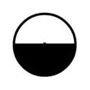How to solve this question?
The rule here is that the white slice is growing by one-eighth of a circle (i.e. 45 degrees) clockwise and anticlockwise alternately. This means that the next shape should have a new white slice added to the left of that shown in the previous shape, which makes up a half white circle.

Question #2What is the next shape in this series?
The correct answer is: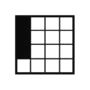How to solve this question?
In this series, there are two black inner shapes – a single black square and a group of three black squares. The first rule is that the single black square, which sits on the group’s bottom square in the first shape, moves two places at a time clockwise along the perimeter. The second rule is that the group of three black squares moves left, one place at a time. This means that the next shape will have the single black square on top of the group’s bottom black square.

Question #3What is the next shape in this series?
The correct answer is: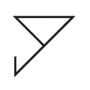How to solve this question?
The first rule here is that the shape turns upside down (180 degrees clockwise) at a time. The second rule here is that one part of the line making up the shape is deleted every step, starting from the top-right corner (in the original shape).

Question #4What is the next shape in this series?
The correct answer is: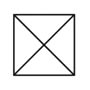How to solve this question?
Each consecutive shape includes the unique forms of the two previous shapes. To illustrate this rule, the third shape has a circle and a big X, which are the only two forms that don’t appear in both the first and second shapes. Therefore, the next shape must have an X and a square, as they are the only two shapes that don’t appear in both previous shapes.

Question #5What is the next shape in this series?
The correct answer is: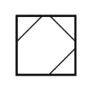How to solve this question?
Here we have four angles that are aligned in the original shape (i.e. top, right, bottom and left angles all meeting in the middle). The left and bottom angles have a black triangle at their edge. The first rule is that the left angle moves horizontally to the right. The second rule is that the top angle moves vertically up (away from the middle). The third rule is that the right angle is moving horizontally to the right (away from the middle). The fourth rule is that the bottom angle is moving vertically up. Only the last answer shape matches all the rules above.

Question #6What is the next shape in this series?
The correct answer is: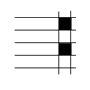How to solve this question?
The number of vertical lines decreases by one and the number of horizontal lines increases by one every step. The black squares fill the intersections between vertical and horizontal lines, similar to a chessboard.

Question #7What is the next shape in this series?
The correct answer is: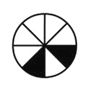How to solve this question?
The top black slice (in the first shape) moves clockwise. The number of places it moves each time follows an arithmetical sequence with a common difference of 2 (i.e. 2, 4, 6, … ). The second (or lower) black slice also moves clockwise. The number of places it moves each time also follows an arithmetical sequence with a common difference of 2 and starting at 1 (i.e. 1, 3, 5, … ). This means that in the next shape, the top black slice (in the first shape) will be eight places away clockwise from its previous position and the second black slice (in the first shape) will be seven places away clockwise from its previous position.

Question #8What is the next shape in this series?
The correct answer is: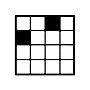How to solve this question?
The entire square turns 45 degrees (one-eighth of a circle) clockwise. The top black square, in the first shape, moves along the perimeter clockwise. The number of places it moves each time follows an arithmetical sequence with a common difference of 2 and starting at 2 (i.e. 2, 4, 6, … ). The bottom black square, in the first shape, moves also along the perimeter clockwise. The number of places it moves each time follows an arithmetical sequence with a common difference of 1 and starting at 1 (i.e. 1, 2, 3, … ).

Share on other sites

Aptitude Test Sample Test (VERBAL)

Soy- and rice- based diets are very popular in Third World countries. The soy bean has a higher phytate content than any other grain or legume that has been studied. Phytate is an organic acid, present in the bran or hulls of all seeds, which blocks the uptake of essential minerals – calcium, magnesium, iron and especially zinc – in the intestinal tract.

Soy beans seem to be highly resistant to many phytate-reducing techniques such as long, slow cooking. Only a long period of fermentation will significantly reduce the phytate content of soy beans. Thus, fermented products such as tempeh and miso provide nourishment that is easily assimilated. When precipitated soy products are consumed with meat, the mineral-blocking effects of the phytates are reduced.

Question # 1

Food made from soy beans is unhealthy

• True
• False
• Can’t say

The correct answer is: False

How to solve this question?
The passage states that the phytate content of the soybean blocks the uptake of essential minerals in the body. This does not necessarily imply that soy foods are unhealthy in themselves, even though the consumption of untreated soybean does affect the uptake of minerals. Phytate can be reduced by some techniques, and when precipitated soy products are consumed with meat, the mineral-blocking effects of phytate are reduced. The statement is not supported by the evidence, and the answer is “False”.

Question # 2

Fermented products have high levels of calcium, magnesium, iron and zinc

• True
• False
• Can't say

The correct answer is: Can't say

How to solve this question?
The passage says only that fermented products such as tempeh and miso provide nourishment that is easily assimilated, but there is no information about their levels of minerals. The passage does not enable this statement to be made, and the answer therefore is “Can’t say”.

Question # 3

Legumes contain phytate

• True
• False
• Can't say

The correct answer is: True

How to solve this question?
The information given in the passage is that soybean has a higher phytate content than any other legume that has been studied; soybean, therefore, is a legume. Because it contains phytate and is a legume, the response to the statement is “True”.

Question # 4

People in Third World countries have low levels of calcium, magnesium, iron and zinc

• True
• False
• Can’t say

The correct answer is: Can’t say

How to solve this question?
Even though phytate blocks the uptake of these minerals, there is no evidence given that people in third-world countries have low levels of these compounds. The passage says that soy- and rice-based diets are very popular in these countries, but it does not go far enough to be able to say definitively that persons are deficient in these essentials. Even if the statement is true, it is not supported by the information in the passage, and the response is “Can’t say”.

-----------------------------------------------------------------------------------------------------------------------------------------------------------------

Business statistics is a scientific approach to decision-making in the face of risk and is used in many disciplines, such as financial analysis, econometrics, auditing, production and operations, and marketing research. The main objective of business statistics is to make inferences about certain characteristics of a population based on information contained in a random sample from the entire population.

Statistical forecasting concentrates on using the past to predict the future by identifying trends, patterns and business drives within the data to develop a forecast. This forecast is referred to as a statistical forecast because it uses mathematical formulas to identify the patterns and trends while testing the results for mathematical reasonableness and confidence. Statistical forecasting is used for forecasting sales and budget.

Question # 5

Using statistics in decision-making eliminates business risks

• True
• False
• Can't say

The correct answer is: Can't say

How to solve this question?
The information given is that business statistics are used for decision-making in the face of risk. There is no suggestion in the passage that such use eliminates business risks, and the answer therefore is “Can’t say”.

Question # 6

Statistical inference refers to extending the knowledge obtained from a random sample from the entire population to the whole population

• True
• False
• Can't say

The correct answer is: True

How to solve this question?
Business statistics are produced by using information obtained in a random sample from the entire population. Inferences that are drawn from this information are used to identify patterns and trends in a forecast that will apply to the whole population. Hence, the answer is “True”.

Question # 7

Statistical forecasting deals with estimating the likelihood of an event taking place in the future, based on available data

• True
• False
• Can’t say

The correct answer is: True

How to solve this question?
The passage says that statistical forecasting concentrates on using the past to identify patterns and trends. This information is used to predict the future, such as forecasting sales and budget. Therefore, the answer is “True”.

Question # 8

Statistical forecasting cannot be used for production and operations

• True
• False
• Can't say

The correct answer is: Can't say

How to solve this question?
The passage says that statistical forecasting is used for forecasting sales and budget, and it does not mention production and operations. This does not mean that statistical forecasting cannot be used for production and operations. Therefore, the response is “Can’t say”.

Share on other sites

Aptitude Test Sample Test (MECHANICAL)

Question #1Two men are carrying a heavy box on a plank. Which man is carrying more of the load?

• Man A
• Man B
• They each carry the same share of the load

The correct answer is: Man B

How to solve this question?
If the heavy box were at the centre of the plank, each man would carry the same share of the load. As the load shifts, so does the load distribution on the plank. As a result, the more the load is distributed towards man B, the more he will need to carry. Imagine the load moving all the way to the right – that would cause man B to carry all of the heavy box and half of the plank, while man A would only carry half the plank.

Question #2You put the same amount of weight at the end of these two pendulums. If you let go of the two pendulums at the same time, which will swing faster?

• A
• B
• No difference

The correct answer is: A

How to solve this question?
When other things are equal, a pendulum with a shorter string swings faster. You can try this out for yourself with some string and a weight. Therefore, the correct answer is A.

Question #3If the shopping trolley was moving in the direction of the big arrow and then you stopped it suddenly, which way would the bottle fall?

• Direction A
• Direction B
• Can’t say

The correct answer is: Direction A

How to solve this question?
The correct answer is direction A. This is because of two mechanical principles. First, objects in motion tend to stay in motion. The bottle was moving in the direction of the big arrow, so it would tend to continue moving in that direction. It might slide in that direction, but its bottom is standing on the trolley, and there is some friction that helps hold it where it is. So rather than slide, it falls over.

Question #4If gear A turns 5 times, how many times will gear B turn?

• 1 time
• 5 times
• 10 times
• 20 times

The correct answer is: 20 times

How to solve this question?
Since gear A is bigger, you know that gear B will turn faster. But you have to count the number of teeth on the gears to find out how much faster. Gear A has 40 teeth and Gear B has 10 teeth. So, every time gear A turns once, gear B turns 4 times. The question says that gear A turns 5 times, so gear B must tum 4 times that much, or 20 times. The correct answer is 20 times.

Question #5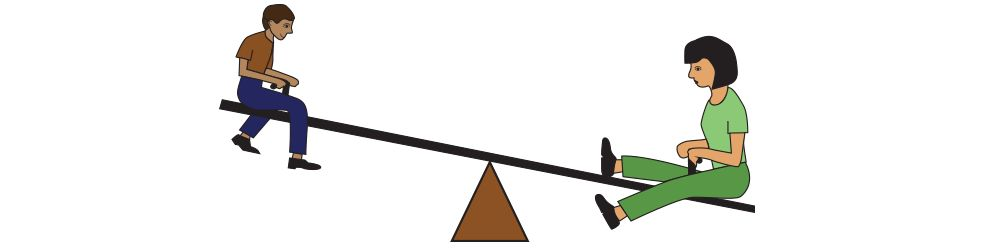A mother weighs 100 Kg and her son weighs 50 Kg. If the son is sitting 6 meters from the pivot, how many metres from the pivot should the mother sit to balance the seesaw?

• 1 metres
• 2 metres
• 3 metres

The correct answer is: 3 metres

How to solve this question?
The mother weighs twice as much as the son, so she has to sit half as far from the pivot to balance the seesaw. Remember, unequal weights balance only if weight times distance is equal. For the son, weight times distance is 50kg x 6 metres equals 300 metres, so for the mother, weight times distance has to be 100kg x 3 metres equals 300 metres to ensure the seesaw is balanced. Therefore, the correct answer is 3.

Question #6If a car and a bowling ball went off a 100-metre cliff at the same time, which would hit the ground first?

• Car
• Ball
• No difference

The correct answer is: No difference

How to solve this question?
Even though the car is much heavier than the bowling ball, both will fall at the same rate. (The resistance of the air is not important here.) The rate of fall is the same, no matter what the weight of the object. Therefore, the correct answer is no difference.

Question #7In what direction will gear 3 turn?

• Clockwise
• Anticlockwise
• Can’t say

The correct answer is: Anticlockwise

How to solve this question?
When there are three gears, the last gear will turn in the same direction as the first. This is true for any odd number of gears. If you have an odd number of gears, the last gear turns in the same direction as the first gear. Therefore, the correct answer is Anticlockwise.

Question #8How much force (in kg) do we need to use to lift the 120 kg weight?

• 10 kg
• 12 kg
• 20 kg
• 24 kg

The correct answer is: 24 kg

How to solve this question?
There are five sections of rope holding up the weight of 120 kg. Three sections of rope go to the lower pulley on the left and two sections of rope go to the lower pulley on the right. Following the general rule we divide the total weight by the number of rope sections supporting this weight: 120 kg / 5 sections of rope is 24. Therefore, the correct answer is 24 kg.

Share on other sites

And for the Personality Test Sample, I am just making a test online in the Online Test Preparation Section. Please wait I will add the test link here tonight or you can keep visit Online Test Preparation Section for updates.

Edited by Xaib Aslam

Share on other sites

AOA,

I know that this is not the right place to post it but i was getting irritated by the irresistable querry about the SMS that i have recently got from NTS asking to fill up BIO-DATA form and  send it to its headquarters after the shorthand/typing test that i have given today.

ANY ONE KNOW THAT WHAT ITS MEANT TO BE ?

#XAIB #MUSA  brother in arms, suggestion required.Yes you are rite that this is not the rite place for further issues you need to open your topic in Other Project Questions / Queries

And I have moved your reply in this section Other Project Questions / Queries and you need to come there and talk about your issue.

Share on other sites

dear admin please guide me that whether calculator is permitted in psychometric test or not?

Well calculator is not allowed and they will give you those questions which can be solved without calculator.

Share on other sites

And for the Personality Test Sample, I am just making a test online in the Online Test Preparation Section. Please wait I will add the test link here tonight or you can keep visit Online Test Preparation Section for updates.